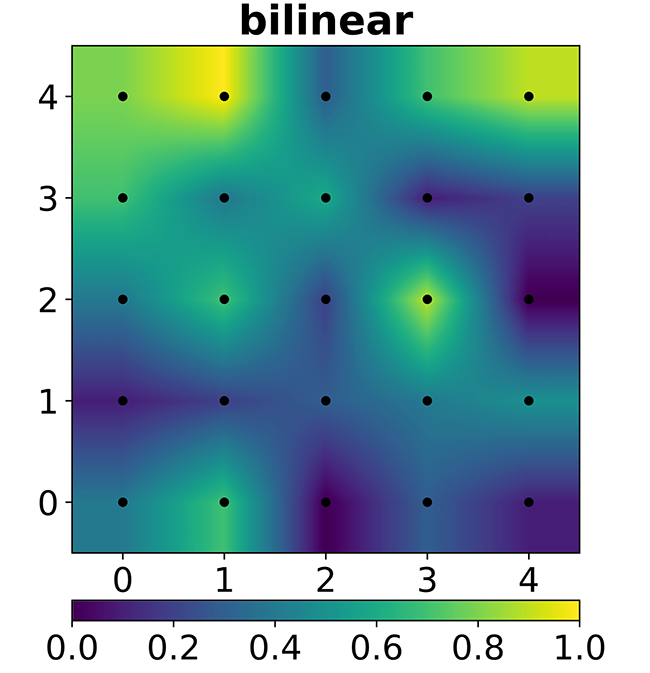# JPEG Image Scaling Algorithms

The Joint Photographic Experts Group (JPEG) format is one of the most common image formats used. It is a compressed image format, that uses a lossy compression algorithm. That means that with a higher compression level, more details in the image are lost. The reason for compressing the image is in order to minimize the file size. For bandwidth hungry users, that means faster download of image content from the web. The trade off between high resolution quality is a loss of detail the higher the compression ratio used.

Photographic images taken with cameras are susceptible to image quality loss when compressed. This is because the graphics generated by the electronic sensor is a raster image. The raster image is constructed from a bitmap, which represents images as dots or picture elements aka pixels. Upscaling the image increases the size, but also leads to a loss of quality when using JPEG formats.

The JPEG format can also be scaled using an image editing software application. Scaling is either up (increase resolution) or down (decrease the resolution). Increasing resolution requires increasing the number of pixels on the width (horizontal) and height (vertical) of the image, measured as:

`resolution = width x height`

A camera that shoots 32 MP (Megapixels) or 32 Million pixels would have an image scale that is approximately:

`resolution = 6464 x 4864 = 31,440,896 pixels`

Cameras listed as 32 MP may not exactly be 32, but in terms of precision it is just an approximate. It also depends on the camera manufacturer’s specs regarding how many pixels on the horizontal and vertical scale that is approximated to the advertised resolution value.

Image scaling is another way of resizing an image. Another term used for resizing is resampling. However take note, they are not necessarily the same thing. Resizing allows you to simply scale up or down the image, without requiring any special processing. Resampling uses resizing but also a more complex algorithm to get the result.

For example, this Python code uses the PIL library module to resize an image and maintain its aspect ratio.

`basewidth = 3000img = Image.open('<name of image file>')wpercent = (basewidth/float(img.size))hsize = int((float(img.size)*float(wpercent)))img = img.resize((basewidth,hsize), Image.ANTIALIAS)`

The basewidth indicates the number of pixels on the horizontal (width). In this example I use 3000 pixels. What the algorithm will do is adjust the height proportional to the width, in order to maintain the aspect ratio. It also performs an antialiasing method to reduce jagged edges or the staircase effect. This a rather simple resizing of the image, but what if you want more quality or better looking results? This is when you apply scaling algorithms by resampling the image.

Using OpenCV, the method to scale or resample an image:

`img = cv2.imread('<name of image file>')cv2.resize(img,fx=scaleX,fy=scaleY, interpolation = cv2.INTER_CUBIC)`

This is just a code snippet sample. It demonstrates resizing an image using a bicubic interpolation algorithm.

## JPEG Scaling Techniques

These are three common types of scaling algorithms used with digital images.

Nearest Neighbor Scaling— This is the fastest and simplest to implement. This technique replaces every pixel with the nearest pixel in the output. When upscaling an image, multiple pixels of the same color will be duplicated throughout the image. For example let us say we have an image region of 2x2 blue pixels. When we upscale it to 3x3, we create 5 additional pixels, that have no color associated with it. Using Nearest Neighbor, the algorithm merely uses the blue pixel’s color to assign to the new pixels. One problem this can introduce are jagged edges or aliasing, so refining the process is required.

Bilinear — This interpolates pixels much better than Nearest Neighbor. It uses the same approach but the computation is much more complex. The technique will set the color or gray value of each pixel according to the nearest pixels. It takes a 2x2 or 4 pixel sample of the 4 nearest cells of the grid, and applies weights based on distance. Bilinear is not continuous over square boundaries, so the colors are not radially symmetric.This example shows how bilinear is not continuous over square boundaries, thus not appearing radially symmetric. (Source Wikipedia)

Bicubic Interpolation — This technique uses interpolation on a 2D grid of pixels. This is a much slower algorithm, since it takes time to process when resampling an image. Bicubic samples 4x4 or 16 pixels at a time. The results are much smoother looking images with less artifacts. This algorithm produces the best results out of the three discussed.

## Resampling Examples

Here are three resamplings of the following photo.

The first sample is 2048 pixels (width) using Nearest Neighbor. 930% magnification.

The second sample is 2048 pixels (width) using Bilinear. 930% magnification.